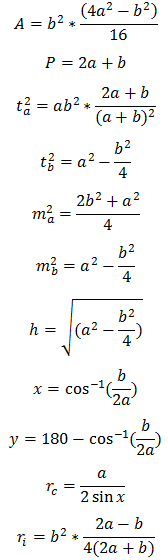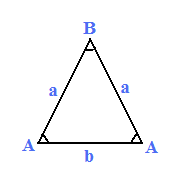# Isosceles Triangle Calculator

This CalcTown calculator calculates the various parameters related to an isosceles triangle.

#### Result

m2
m
m
m
m
m
m
degrees
degrees
m
mWhere,

a = length of one of the two equal sides

b = length of third side

A = area of the isosceles triangle

P = perimeter of the triangle

ta = angle bisector of side a

tb = angle bisector of side b

ma = median of side a

mb = median of side b

h = height of the isosceles triangle

x = the equal angle of the isosceles triangle

y = third angle of the isosceles triangle

ri = inscribed circle radius of the isosceles triangle

rc = circumscribed circle radius of the isosceles circle